The difference between a Spreadsheet and a Worksheet is that an Excel Spreadsheet file contains Worksheets that some people call “tabs”. See screenshot below.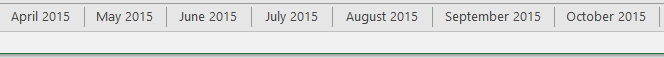To Unprotect Worksheet(s) all you need to do is to follow this guide:

2. Press ALT+F11 to open VBA. You will then see all your worksheets on the left hand side: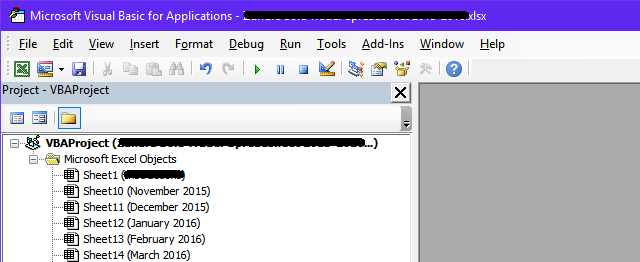3. Double click on any of the worksheets and paste the following code:

```Sub PasswordBreaker()
Dim i As Integer, j As Integer, k As Integer
Dim l As Integer, m As Integer, n As Integer
Dim i1 As Integer, i2 As Integer, i3 As Integer
Dim i4 As Integer, i5 As Integer, i6 As Integer
On Error Resume Next
For i = 65 To 66: For j = 65 To 66: For k = 65 To 66
For l = 65 To 66: For m = 65 To 66: For i1 = 65 To 66
For i2 = 65 To 66: For i3 = 65 To 66: For i4 = 65 To 66
For i5 = 65 To 66: For i6 = 65 To 66: For n = 32 To 126
ActiveSheet.Unprotect Chr(i) & Chr(j) & Chr(k) & _
Chr(l) & Chr(m) & Chr(i1) & Chr(i2) & Chr(i3) & _
Chr(i4) & Chr(i5) & Chr(i6) & Chr(n)
If ActiveSheet.ProtectContents = False Then
MsgBox "One usable password is " & Chr(i) & Chr(j) & _
Chr(k) & Chr(l) & Chr(m) & Chr(i1) & Chr(i2) & _
Chr(i3) & Chr(i4) & Chr(i5) & Chr(i6) & Chr(n)
Exit Sub
End If
Next: Next: Next: Next: Next: Next
Next: Next: Next: Next: Next: Next
End Sub```

It should look like this: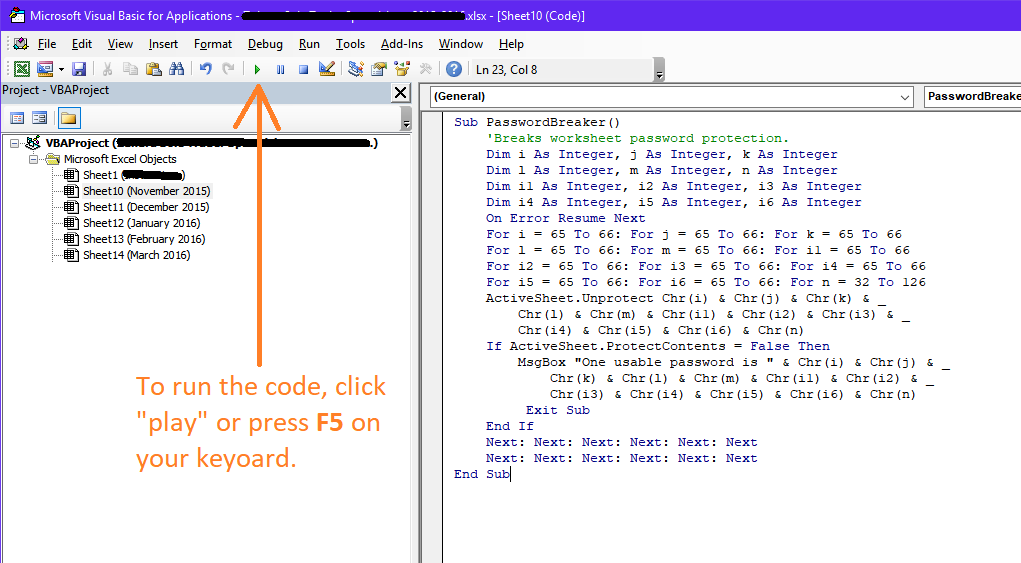4. Cluck Run (“play” button) or press F5 on your keyboard to run the code. This will unprotect the worksheet on which you ran this code.

You will see a small pop up window with the password you need to use to unprotect the remaining worksheets.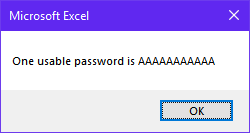5. Use the above password (it will be different in your case, probably something like AAABAABBBBBv) to unprotect any other worksheet that is protected in this Spreadsheet file.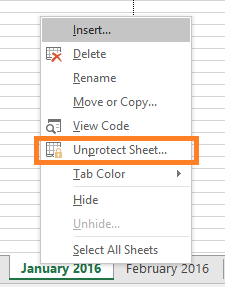Job done. Couldn’t be easier.